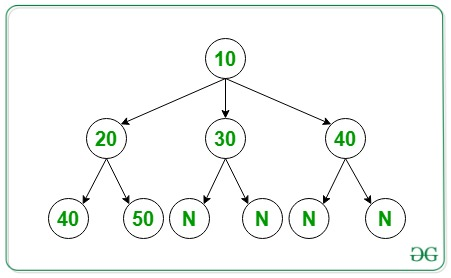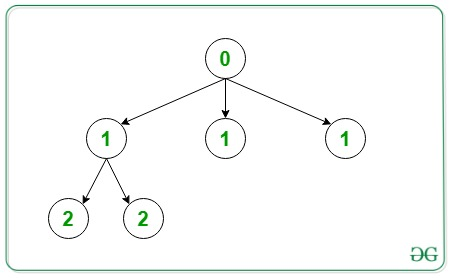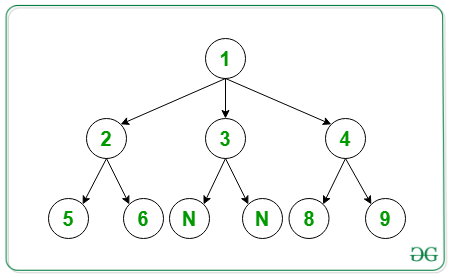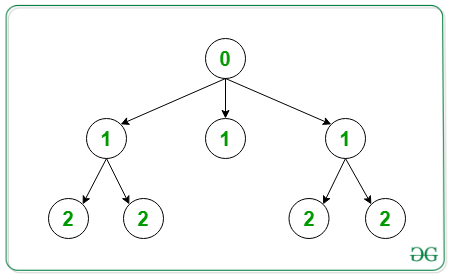# Replace every node with depth in N-ary Generic Tree

Given an array arr[] representing a Generic(N-ary) tree. The task is to replace the node data with the depth(level) of the node. Assume level of root to be 0.

Array Representation: The N-ary tree is serialized in the array arr[] using level order traversal as described below:

• The input is given as a level order traversal of N-ary Tree.
• The first element of the array arr[] is the root node.
• Then, followed by a number N, which denotes the number of children of the previous node. Value zero denotes Null Node.

Examples:

Input: arr[] = { 10, 3, 20, 30, 40, 2, 40, 50, 0, 0, 0, 0 }
Below is the N-ary Tree of the above array level order traversal:Output:
Below is the representation of the above output:Input: arr[] = {1, 3, 2, 3, 4, 2, 5, 6, 0, 0, 2, 8, 9}
Below is the N-ary Tree of the above array level order traversal:Output:
Below is the representation of the above output:## Recommended: Please try your approach on {IDE} first, before moving on to the solution.

Approach:

• Traverse the tree starting from root.
• While traversing pass depth of node as a parameter.
• Track depth by passing it as 0 for root and (1 + currrent level) for children.

Below is the implementation of the above approach:

 `// C++ program to implement node with ` `// it's depth value ` `#include ` `using` `namespace` `std; ` ` `  `// Treenode class using template ` `template` `<``typename` `T> ` `class` `TreeNode { ` `public``: ` `    ``// To store node value ` `    ``T data; ` ` `  `    ``// Pointer to TreeNode to store ` `    ``// the child node ` `    ``vector*> children; ` ` `  `    ``// Constructor to assign data ` `    ``// to node ` `    ``TreeNode(T data) ` `    ``{ ` `        ``this``->data = data; ` `    ``} ` ` `  `    ``// Destructors to delete a node ` `    ``~TreeNode() ` `    ``{ ` `        ``for` `(``int` `i = 0; ` `             ``i < children.size(); i++) { ` `            ``delete` `children[i]; ` `        ``} ` `    ``} ` `}; ` ` `  `// Function to take input level wise ` `// i.e., in level order traversal ` `TreeNode<``int``>* takeInputLevelWise(``int` `arr[]) ` `{ ` `    ``int` `idx = 1; ` ` `  `    ``// Input root ` `    ``int` `rootData = arr; ` ` `  `    ``// Initialize tree with a root node ` `    ``TreeNode<``int``>* root ` `        ``= ``new` `TreeNode<``int``>(rootData); ` ` `  `    ``// Intialise queue for appending ` `    ``// node as a child of parent in ` `    ``// N-ary tree ` `    ``queue*> pendingNodes; ` ` `  `    ``// Push the root node in queue ` `    ``pendingNodes.push(root); ` ` `  `    ``// While queue is not empty append ` `    ``// child to the root ` `    ``while` `(pendingNodes.size() != 0) { ` ` `  `        ``// Take the first node ` `        ``TreeNode<``int``>* front ` `            ``= pendingNodes.front(); ` `        ``pendingNodes.pop(); ` ` `  `        ``// Input number of child ` `        ``int` `numChild = arr[idx]; ` `        ``idx++; ` ` `  `        ``for` `(``int` `i = 0; i < numChild; i++) { ` ` `  `            ``int` `childData = arr[idx]; ` `            ``idx++; ` ` `  `            ``// Make child Node ` `            ``TreeNode<``int``>* child ` `                ``= ``new` `TreeNode<``int``>(childData); ` ` `  `            ``// Append child node to ` `            ``// it's parent ` `            ``front->children.push_back(child); ` `            ``pendingNodes.push(child); ` `        ``} ` `    ``} ` `    ``return` `root; ` `} ` ` `  `// Function to print each node data ` `// in level order ` `void` `printLevelATNewLine(TreeNode<``int``>* root) ` `{ ` `    ``queue*> q; ` `    ``q.push(root); ` `    ``q.push(NULL); ` `    ``while` `(!q.empty()) { ` ` `  `        ``TreeNode<``int``>* first = q.front(); ` `        ``q.pop(); ` ` `  `        ``if` `(first == NULL) { ` `            ``if` `(q.empty()) { ` `                ``break``; ` `            ``} ` `            ``cout << endl; ` `            ``q.push(NULL); ` `            ``continue``; ` `        ``} ` ` `  `        ``cout << first->data << ``" "``; ` ` `  `        ``for` `(``int` `i = 0; ` `             ``i < first->children.size(); i++) { ` `            ``q.push(first->children[i]); ` `        ``} ` `    ``} ` `} ` ` `  `// Helper function to replace the ` `// node data with their level value ` `void` `helper(TreeNode<``int``>* root, ` `            ``int` `depth) ` `{ ` ` `  `    ``// Replace the node data with ` `    ``// it's depth ` `    ``root->data = depth; ` `    ``for` `(``int` `i = 0; ` `         ``i < root->children.size(); i++) { ` ` `  `        ``helper(root->children[i], depth + 1); ` `    ``} ` `} ` ` `  `// Function to replace with depth ` `void` `replaceWithDepthValue(TreeNode<``int``>* root) ` `{ ` `    ``helper(root, 0); ` `} ` ` `  `// Driver Code ` `int` `main() ` `{ ` ` `  `    ``// Given level order traversal in ` `    ``// the array arr[] ` `    ``int` `arr[] = { 10, 3, 20, 30, 40, 2, ` `                  ``40, 50, 0, 0, 0, 0 }; ` ` `  `    ``// Intialise Tree ` `    ``TreeNode<``int``>* root; ` `    ``root = takeInputLevelWise(arr); ` ` `  `    ``// Function call to replace with ` `    ``// depth value ` `    ``replaceWithDepthValue(root); ` ` `  `    ``// Function call to print ` `    ``// in level order ` `    ``printLevelATNewLine(root); ` `    ``return` `0; ` `} `

Output:

```0
1 1 1
2 2
```

Time Complexity: O(N), where N is the number of nodes in Tree.
Auxiliary Space: O(N), where N is the number of nodes in Tree.

Attention reader! Don’t stop learning now. Get hold of all the important DSA concepts with the DSA Self Paced Course at a student-friendly price and become industry ready.

My Personal Notes arrow_drop_upCheck out this Author's contributed articles.

If you like GeeksforGeeks and would like to contribute, you can also write an article using contribute.geeksforgeeks.org or mail your article to contribute@geeksforgeeks.org. See your article appearing on the GeeksforGeeks main page and help other Geeks.

Please Improve this article if you find anything incorrect by clicking on the "Improve Article" button below.

Article Tags :
Practice Tags :

1

Please write to us at contribute@geeksforgeeks.org to report any issue with the above content.